# Automate from Excel: Probabilistic Analysis

To automate a Probabilistic Analysis:

1. Create a new UnWedge model file.
2. Set up the Project Settings dialog. Select Analysis > Project Settings.
3. Set Analysis Type = Probabilistic .
4. Set the Sampling Method and Number of Samples .
5. Select OK to close the dialog.
6. Set up the Probabilistic Input Data dialog. Select Analysis > Input Data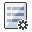.
7. Under the General tab, set the checkbox controls as applicable.
8. Under the Joint Orientations tab, add the Joints and set their Properties as applicable.
• If you define exactly 3 Joints, this will form only one possible joint combination. All 3 joint orientations are automatable from the Excel template.
• If you define more than 3 Joints, automation will be performed assuming all possible joint combinations. The joint orientations are not automatable, and any joint orientations input into the Excel template will be ignored.
9. Under the Joint Properties tab, add Properties and set their checkbox and combo box controls as applicable.
• If you define more than 3 Properties, the joint properties are not automatable, and any joint orientations input into the Excel template will be ignored.
• Ground/Truncation Surface and Water Pressure Grid are not automated inputs from Automate from Excel. If any water pressure surface or grid are to be applied, they must be defined in the model.
10. Select OK to close the dialog.
11. Set up the Field Stress dialog. Select Analysis > Field Stress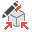.
12. Set the checkbox and combo box controls as applicable.
13. Set the Advanced Settings for Stresses as applicable.
14. Set up the Scale Wedges dialog. Select Analysis > Scale Wedges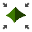.
15. Set the checkbox controls as applicable.
16. Set Wedge to Scale = All Wedges. Automated scaling will be applied to all 10 possible wedges.
17. Assign statistical variables in the Statistics menu.
18. Pre-process input data.
1. Save a copy of the input Excel template.
2. The Excel workbook is protected to prevent accidental manipulation of worksheets. The predefined column header cells are protected to prevent accidental manipulation of input columns.
• Each row of input data represents a separate Probabilistic computation in UnWedge (i.e., number of analysis = number of samples).
3. Enter in all applicable numerical data corresponding to the selections in the Probabilistic Input Data dialog, Field Stress dialog, and Scale Wedge dialog.
4. Enter in all applicable statistical values corresponding to selections in the Joint Orientations Statistics dialog, Joint Properties Statistics dialog, and Field Stress Statistics dialog.
5. All applicable data must be entered into the Excel input file. If any required columns are left blank, in an incorrect format, or outside the valid range, the Error Log generated from Automate from Excel will indicate these errors.
6. Save and close the Excel input file.
7. Select Automate from Excel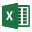from the File menu.
8. In the Automated Compute dialog:
9. Add Excel input file(s) to the File Queue by selecting the Open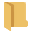button.
10. Only created files from UnWedge Automation Template_Probabilistic.xlsx can be automated since the project setting and input data setup, and file reading is format-specific to the program UnWedge and to the Analysis Type.
11. Select the Compute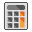button to run the analysis and write the results on all Excel input files in the File Queue.
• If there is only 1 Joint Combination, one Excel file per analysis case (i.e., row) of the Excel input file is generated; the results are separated by columns and listed as one tunnel analysis (i.e. sample) per row. Each output file contains number of rows = number of samples.
• If there are multiple Joint Combinations, one Excel file per analysis case (i.e., row) of the Excel input file is generated; the results are separated by columns and listed as one joint combination per subsample, per row. Each output file contains number of rows = number of samples (approximately). E.g., if there were 5 possible joint combinations and 100 samples, you would have 20 samples of each joint combination.
12. Check the Error Log for any errors detected in the Excel input file. If any errors are reported, correct them in the Excel input file and repeat step 9.
13. Post-process output data.